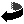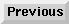# Going Nowhere with Nobody

I wanted to find good examples of linked lists in Python on the net. Nothing really stood out to me so I just wrote a more complete example based of some others:

``````import logging
from logging.config import fileConfig

fileConfig('logging.ini')
logger = logging.getLogger()

class Node:
def __init__(self, cargo=None, next=None):
self.cargo = cargo
self.next = next

def __str__(self):
return str(self.cargo)

def pretty_print(self):
if self.next != None:
node = self.next
node.pretty_print()
if ord(self.cargo) % 16:
print(f"\t{self.cargo}\t", end="")
else:
print(f"\n{self.cargo}")

def __init__(self):
self.length = 0

def print_list(self):

node = Node(cargo)
self.length = self.length + 1

def search(self, search_node):
node_count = 0
logger.debug(f"Started here: {current}")
while current != None:
if current.cargo == search_node:
print(f"Done! Saw {node_count} node(s))")
return current
current = current.next
node_count += 1

def delete(self, node):
if node == None or node.next == None:
return False
next = node.next
node.cargo = next.cargo
node.next = next.next
return True

Note too the use of the `logging` module. Read up on that @ PSF (python.org)page last modified: 2020-04-12